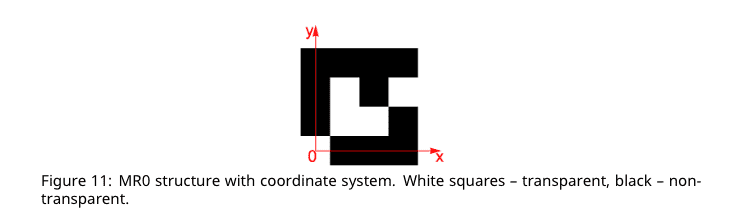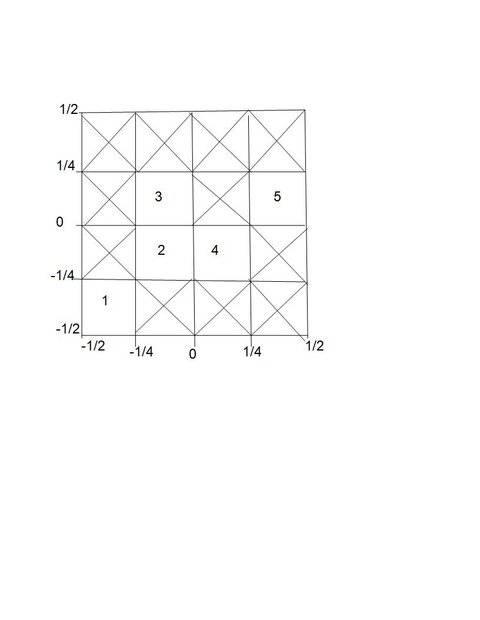# Intensity of central maxima of crystal diffraction

• phantomvommand
In summary, when the crystal above is illuminated with light of intensity ##I_0##, the intensity at the central maximum is ##(\frac {5} {16} )^2 I_0##. This can be explained using Fraunhofer diffraction theory, where the complex amplitude of the diffracted wave is dependent on the transparent area of the unit cell. The intensity at the center is proportional to the total number of sources and the solid angle of the diffraction maximum, resulting in a peak intensity of ##I_0\frac{(5)^2}{(16)^2}##.f

#### phantomvommand

Homework Statement
Relevant Equations
Intensity proportional to Amplitude of E-field squared.When the crystal above is illuminated with light of intensity ##I_0##, what is the intensity at the central maximum? (The picture shown above is a 4 x 4 unit cell of the crystal)
The answer is ##(\frac {5} {16} )^2 I_0##. Why?

Apparently, Electric field is proportional to the transparent area, and will be maximum if the whole unit cell is transparent. I feel that it should be intensity that's proportional to the transparent area instead?
I cannot see how amplitude of electric field is related to transparent squares.

Thanks for all the help.

From diffraction theory, the intensity of the primary maxima for ##N ## sources (in phase), (or for a single slit diffraction pattern made from ## N ## sources), is proportional to ## N^2 ##, because the resultant electric field amplitude ## E ## is proportional to ## N ##. The reason energy is conserved is that for ## N ## sources, the angular width of the diffraction pattern (central maximum) ## \Delta \theta \approx \frac{\lambda}{b} ##, where ## b ## is the width of the ## N ## sources, (e.g. arranged in a square), so that the solid angle of the maximum, which is proportional to ## (\Delta \theta )^2 ##, is inversely proportional to the total area of the sources. Thereby the solid angle of the diffraction maximum is inversely proportional to ## N ##. The power is a product of the intensity and the solid angle. It turns out the power in the entire maximum for ## N ## sources will be proportional to ## N ##. The peak goes as ## N^2 ##, but the solid angle spread goes as ## \frac{1}{N} ##.

Note: With circular symmetry, (with a circular source of diameter ## d ##), the ## \Delta \theta ## of the diffraction pattern, which is an Airy disc pattern, is once again proportional to ## \frac{\lambda}{d} ##, so that the solid angle of the diffraction maximum is again inversely proportional to the area of the source.

Last edited:
•phantomvommand and hutchphd
And the "book" answer is that the forward amplitude is proportional to the corresponding (0,0) Fourier component which is just the normalized integral. But I like the @Charles Link explanation also..

.

•I analyze this problem using Fraunhofer diffraction theory, to whit, the complex amplitude of the diffracted wave is,
$$U(z,y,z)\propto \int \int_{Aperture}A(x^{'},y^{'})e^{-i\frac{2\pi}{\lambda}(ux^{'}+vy^{'})}dx^{'}dy^{'}$$
where ##A(x^{'},y^{'})## is the complex amplitude over the surface of the aperture. I wave my hand and declare the constant of proportionality is ##\sqrt{I_0}##, and refer to the diagram below for the limits of integration in the five transparent regions of the unit cell.$$U_1= \sqrt{I_0}\int_{-\frac{1}{2}}^{-\frac{1}{4}}e^{-i\frac{2\pi}{\lambda}ux^{'}}dx^{'}\int_{-\frac{1}{2}}^{-\frac{1}{4}}e^{-i\frac{2\pi}{\lambda}vy^{'}}dy^{'}$$
$$=\sqrt{I_0}\frac{e^{-i\frac{2\pi}{\lambda}ux^{'}}}{-i\frac{2\pi}{\lambda}u}|_{-\frac{1}{2}}^{-\frac{1}{4}}\frac{e^{-i\frac{2\pi}{\lambda}vy^{'}}}{-i\frac{2\pi}{\lambda}v}|_{-\frac{1}{2}}^{-\frac{1}{4}}$$
$$=\sqrt{I_0}\frac{e^{i\frac{\pi}{4\lambda}(3u+3v)}}{16}sinc(\frac{\pi u}{4\lambda})sinc(\frac{\pi v}{4\lambda})$$
in likewise manner we compute,
$$U_2=\sqrt{I_0}\frac{e^{i\frac{\pi}{4\lambda}(u+v)}}{16}sinc(\frac{\pi u}{4\lambda})sinc(\frac{\pi v}{4\lambda})$$
$$U_3=\sqrt{I_0}\frac{e^{i\frac{\pi}{4\lambda}(u-v)}}{16}sinc(\frac{\pi u}{4\lambda})sinc(\frac{\pi v}{4\lambda})$$
$$U_4=\sqrt{I_0}\frac{e^{i\frac{\pi}{4\lambda}(-u+v)}}{16}sinc(\frac{\pi u}{4\lambda})sinc(\frac{\pi v}{4\lambda})$$
$$U_5=\sqrt{I_0}\frac{e^{i\frac{\pi}{4\lambda}(-3u-v)}}{16}sinc(\frac{\pi u}{4\lambda})sinc(\frac{\pi v}{4\lambda})$$
We sum the individual contributions and find the intensity by taking the dot product of ##U## with its complex conjugate.
$$U=U_1+U_2+U_3+U_4+U_5$$
$$I=U\cdot U^{*}$$
$$=\frac{I_0}{(16)^2}sinc(\frac{\pi u}{4\lambda})^2sinc(\frac{\pi v}{4\lambda})^2[5+4\cos(\frac{\pi}{2}(u+v))+2\cos(\frac{\pi}{2}(u+2v))+2\cos(\frac{\pi}{2}(2u+v))+2\cos(\frac{\pi}{2}(3u+2v))+2\cos(\frac{\pi}{2}(u))+2\cos(\frac{\pi}{2}(v))+2\cos(\frac{\pi}{2}(u-v))2\cos(\frac{\pi}{2}(4u))+2\cos(\frac{\pi}{2}(4u+2v))]$$
We evaluate this at the center of the cell to find ##I=I_0\frac{(5)^2}{(16)^2}##.

#### Attachments

Last edited:
•